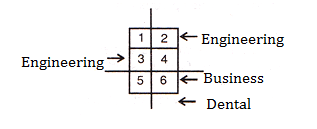Question No 1 to 4: Six square states having equal area in a country are located in North-South directions in two columns next to each other. States are located in the given order, State 1, State 3, and State 5 are on the western side and State 2, State 4, and State 6 are on the eastern side. Within the six states, there are exactly four Dental institutes, two business institutes, and two Engineering institutes. These eight institutions are located as follows:
No institution is in more than one of the states.
None of the states contains more than one business institute, and none contains more than one Engineering institute.
None of the states contains both a business institute and an Engineering institute.
Each business institute is located in a state that contains at least one Dental institute.
The Engineering institutes are located in two states that do not share a common boundary.
State 3 contains an Engineering institute, and State 6 contains a business institute.

Question 1 :  If one of the states contains exactly two Dental institutes and exactly one Engineering institute, then which combinational of three states might contain no Dental institute?
A. 1, 4, 5
B. 2, 3, 5
C. 2, 4, 6
D. 1,3, 5
E. 4, 5, 6

Question 2 : Which one of the following could be true?
A. State 1 contains exactly one Engineering institute
B. State 2 contains exactly one business institute
C. State 5 contains exactly one Engineering institute
D. State 6 contains exactly one Engineering institute
E. State 1 contains exactly one Dental institute

Question 3 : A complete and accurate list of the states, any one of which could contain the business
institute that is not in State 6, would be________.
A. 1, 4
B. 2, 4
C. 1, 4, 5
D. 4, 5
E. 1, 2, 4, 5

Question 4 :  If each of the six states contains at least one of the eight institutions, then which one of the following must be true?
A. There is business institute in state 1
B. There is a Dental institute in state 2
C. There is a Dental institute in state 3
D. There is a Dental institute in state 4
E. There is a business institute in state 4

Solution For Questions 1 to 4: State 3 contains Engineering institute and state 6 contains a business institute. State 6 cannot contain an Engineering institute and also states 1, 4 and 5 cannot contain an Engineering institute as they share the common boundary. Therefore, state 2 contains an Engineering institute. From the given information and above conclusions the following diagram can be drawn.